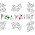## Thursday, March 28, 2013

### TETRIS

graphsize 600,440
fastgraphics
font ("arial",20,100)
Dim i(4):Dim j(4):Dim l(4):Dim o(4):Dim s(4):Dim t(4):Dim z(4)
i = { 0x10F00, 0x12222, 0x100F0, 0x14444}
j = { 0x144C0, 0x18E00, 0x16440, 0x10E20}
l = {0x14460, 0x10E80, 0x1C440, 0x12E00 }
o = { 0x1CC00, 0x1CC00, 0x1CC00, 0x1CC00}
s = {0x106C0, 0x18C40, 0x16C00, 0x14620 }
t = {0x10E40, 0x14C40, 0x14E00, 0x14640}
z = { 0x10C60, 0x14C80, 0x1C600, 0x12640}
Dim board(14,29)
Dim stat(7)
Score = 0
endgame = false
initialwait= 0.1
# main loop for each newpiece
do
wait = initialwait
rot=int(rand*4)
shape = int (rand*7)
stat[shape]=stat[shape]+1
cores = int (rand*7)+1
piecedown = false
ypos=-1
xpos=5
# loop for each frame
do
ypos=ypos+1
# controls
for press = 1 to 4
a = key
if 16777234=a then xpos=xpos -checkmove(ref (board),a,xpos,ypos,shape\$)
if 16777236 = a then xpos=xpos +checkmove(ref (board),a,xpos,ypos,shape\$)
if 16777237=a then wait =0.01
if 16777235=a then
oldrot=rot
rot = rot+1
if rot = 4 then rot = 0
gosub selectshape
if checkmove(ref (board),a,xpos,ypos,shape\$)=0 then
rot = oldrot
gosub selectshape
end if
end if
pause wait
next press
# procedures
gosub scorepanel
gosub selectshape
gosub drawboard
gosub drawshape
refresh
until piecedown = true
# these procedures are executed when a piece is down
gosub newboard
gosub takeline
gosub takeline
until endgame = true
#last instructions before end of game
Color white
font ("arial",50,100)
text 70,100, "Game Over"
refresh
end
scorepanel:
color darkgreen
rect 0,0,600,450
color green
Text 300,20,"Use arrow keys"
Text 300,60, "I .... "+stat
Text 300,100,"J .... "+stat
Text 300,140,"L .... "+stat
Text 300,180,"O .... "+stat
Text 300,220,"S .... "+stat
Text 300,260,"T .... "+stat
Text 300,300,"Z .... "+stat
Text 300,350,"Score .... "+Score
return
selectshape:
if shape = 0 then shape\$ = tobinary (i[rot])
if shape = 1 then shape\$ = tobinary (j[rot])
if shape = 2 then shape\$ = tobinary (l[rot])
if shape = 3 then shape\$ = tobinary (o[rot])
if shape = 4 then shape\$ = tobinary (s[rot])
if shape = 5 then shape\$ = tobinary (t[rot])
if shape = 6 then shape\$ = tobinary (z[rot])
return
function checkmove (ref (board),a,xpos,ypos,shape\$)
checkmove=1
p=1
For v = 1 to 4
For h = 1 to 4
p=p+1
if mid(shape\$,p,1)="1" then
if board[xpos+h,ypos+v]>0 then checkmove=0
if chr(a)="N" and (xpos+h>11 or xpos+h<2) then
checkmove=0
return
end if
if a=16777236 and xpos+h>10 then checkmove=0
if a=16777236 and board[xpos+h+1,ypos+v]>0 then checkmove=0
if a=16777234 and xpos+h<3 then checkmove=0
if a=16777234 and board[xpos+h-1,ypos+v]>0 then checkmove=0
end if
next h
next v
end function
drawshape:
if cores = 1 then color red
if cores = 2 then color blue
if cores = 3 then color yellow
if cores = 4 then color orange
if cores = 5 then color green
if cores = 6 then color purple
if cores = 7 then color white
p=1
For v = 1 to 4
For h = 1 to 4
p=p+1
if mid(shape\$,p,1)="1" then
rect xpos*20+h*20,ypos*20+v*20,19,19
if board[xpos+h,ypos+v+1]>0 or ypos+v=20 then piecedown=true
end if
next h
next v
if piecedown=true then
if ypos<2 then endgame =true
return
end if
return
newboard:
p=2
For v = 1 to 4
For h = 1 to 4
if mid(shape\$,p,1)="1" then board[xpos+h,ypos+v]=cores
p=p+1
next h
next v
return
drawboard:
For y = 1 to 20
For x = 2 to 11
if board[x,y] = 1 then color red
if board[x,y] = 2 then color blue
if board[x,y] = 3 then color yellow
if board[x,y] = 4 then color orange
if board[x,y] = 5 then color green
if board[x,y] = 6 then color purple
if board[x,y] = 7 then color white
if board[x,y]=0 then color black
rect x*20,y*20,19,19
next x
next y
return
takeline:
For y = 20 to 1 step -1
complete = true
For x = 2 to 11
if board[x,y]=0 then complete = false
next x
if complete = true then
For row = y to 1 step -1
For position = 1 to 10
board[position,row]=board[position,row-1]
next position
next row
Score = Score +1
ix = initialwait*.9
initialwait=ix
end if
next y
return

1.2.